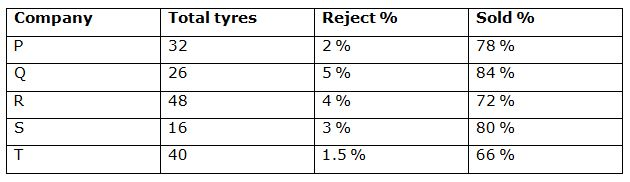# IBPS Clerk Prelims Quantitative Aptitude Questions 2019 (Day-10)

Dear Aspirants, Our IBPS Guide team is providing new series of Quantitative Aptitude Questions for IBPS Clerk Prelims 2019 so the aspirants can practice it on a daily basis. These questions are framed by our skilled experts after understanding your needs thoroughly. Aspirants can practice these new series questions daily to familiarize with the exact exam pattern and make your preparation effective.

Check here for IBPS Clerk Prelims Mock Test 2019

[WpProQuiz 7324]

Directions (Q. 1 – 5): In each of these questions two equations (I) and (II) are given. You have to solve both the equations and give answer as,

a) If x > y

b) If x ≥ y

c) If x < y

d) If x ≤ y

e) If x = y or no relation can be established between x and y

1) I) x = √(12875 – 3271)

II) y = ∛474552

2) I) x2 – 6x – 72 = 0

II) y2 + 3y – 88 = 0

3) I) 3x2 + 26x + 56 = 0

II) 2y2 + 15y + 28 = 0

4) I) 4x – 5y = -12

II) 3x + 4y = 53

5) I) x2 – 17x + 52 = 0

II) y2-5y – 66 = 0

Data interpretation

Directions (Q. 6 – 10) Study the following information carefully and answer the given questions.

The following table shows the total number of tyres (In lakhs) manufactured by 5 different companies in a certain year.6) Total number of tyres rejected by the company P is approximately what percentage more/less than the total number of tyres rejected by the company T?

a) 7 % less

b) 15 % more

c) 15 % less

d) 24 % more

e) 7 % more

7) Find the average number of tyres manufactured by all the given companies together?

a) 32.4 lakhs

b) 28.5 lakhs

c) 30.8 lakhs

d) 34.6 lakhs

e) None of these

8) Find the difference between the total number of tyres sold by the company Q to that of company S?

a) 792600

b) 756800

c) 833200

d) 809270

e) None of these

9) Find the ratio between the total number of tyres rejected by the company Q to that of R?

a) 72: 55

b) 53: 115

c) 84: 137

d) 65: 96

e) None of these

10) Total number of tyres sold by the company P is approximately what percentage of total number of tyres sold by the company R?

a) 60 %

b) 75 %

c) 50 %

d) 85 %

e) 105 %

Directions (1-5):

I) x = √(12875 – 3271)

X = √9604 = 98

II) y = ∛474552

Y = 78

X > y

I) x2 – 6x – 72 = 0

(x – 12) (x + 6) = 0

X = 12, – 6

II) y2 + 3y – 88 = 0

(y + 11) (y – 8) = 0

Y = -11, 8

Can’t be determined

I) 3x2 + 26x + 56 = 0

3x2 + 12x + 14x + 56 = 0

3x(x + 4) + 14(x + 4) = 0

(3x + 14) (x + 4) = 0

X = -14/3, -4 = -4.667, -4

II) 2y2 + 15y + 28 = 0

2y2 + 8y + 7y + 28 = 0

2y(y + 4) + 7 (y + 4) = 0

(2y + 7) (y + 4) = 0

Y = -7/2, -4 = -3.5, -4

X ≤ y

4x – 5y = -12 –> (1)

3x + 4y = 53 –> (2)

By solving the equation (1) and (2), we get,

X = 7, y = 8

X < y

I) x2 – 17x + 52 = 0

(x -14) (x – 3) = 0

X = 14, 3

II) y2 – 5y – 66 = 0

(y – 11) (y + 6) = 0

Y = 11, -6

Can’t be determined

Directions (6-10):

Total number of tyres rejected by the company P

= > 3200000*(2/100) = 64000

Total number of tyres rejected by the company T

= > 4000000*(1.5/100) = 60000

Required % = [(64000 – 60000)/60000]*100 = 7 % more

The average number of tyres manufactured by all the given companies together

= > (32 + 26 + 48 + 16 + 40)/5

= > 162/5 = 32.4 lakhs

The total number of tyres sold by the company Q

= >2600000*(95/100)*(84/100) = 2074800

The total number of tyres sold by the company S

= > 1600000*(97/100)*(80/100) = 1241600

Required difference = 2074800 – 1241600 = 833200

The total number of tyres rejected by the company Q

= > 26*(5/100) = 1.3 lakhs

The total number of tyres rejected by the company R

= > 48*(4/100) = 1.92 lakhs

Required ratio = 1.3: 1.92 = 130: 192 = 65: 96

Total number of tyres sold by the company P

= > 3200000*(98/100)*(78/100) = 2446080

Total number of tyres sold by the company R

= > 4800000*(96/100)*(72/100) = 3317760

Required % = (2446080/3317760)*100 = 73.726 = 75 %